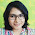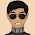Simple Method for writing Lewis Structures for N2O3 | Chemistry Net

# Simple Method for writing Lewis Structures for N2O3

Simple Method for writing Lewis Structures for N2O3

# Simple Method for writing Dot Structures of N2O3

A simple procedure for writing Lewis structures is given in a previous article entitled “Lewis Structures and the Octet Rule”. Several worked examples relevant to this procedure were given in previous posts please see the Sitemap - Table of Contents (Lewis Electron Dot Structures).

Another example  for writing Lewis structures following the above procedure is given below.

How can we construct the N2O3 Lewis structure?

Step 1: Connect the atoms with single bonds. The central atoms are the nitrogen atoms.

Step 2: Calculate the # of electrons in π bonds (multiple bonds) using  formula (1) in the article entitled “Lewis Structures and the Octet Rule”.

Where n in this case is 5 since N2O3 consists of five atoms.

Where V = (5 + 5 + 6 + 6 + 6 ) = 28

Therefore, P = 6n + 2 – V = 6 *5 + 2 – 28 = 4  Therefore, there are 4π electrons in N2O3 and 2 double bonds or a triple bond must be added to the structure in Step 1.

Step 3 & 4: The Lewis structures of N2O3 are as follows:

Another resonance structure of N2O3 is given below but is less plausible compared to the one given in Figure 1 due to large charge separation and the adjacent positive charges.Relevant Posts

Lewis Structures|Octet Rule: A Simple Method to write Lewis Structures

Lewis Dot Structures - Simple Procedure for writing Lewis Structures of Nitrous Oxide (N2O)

Simple method for writing Lewis Structures for N2O4

References

1. G.N. Lewis, J.A.C.S, 38, 762-785, (1916)
2. E. C. McGoran, J. Chem. Educ., 68, 19-23 (1991)
3. A.B.P. Lever, J. Chem. Educ., 49, 819-821, (1972)

Key Terms

resonance structures of nitrous oxide N2O3, Lewis electron structures of dinitrogen trioxide, chemical formula of N2O3, simple procedure for drawing Lewis structures of dinitrogen trioxide,

#### 6 comments:

1.how will we come to know in between which 2 atoms double bond may be present?

1.Thank you for asking! This is a good question.

Multiple bonds are placed between atoms by trial and error. A chemist's intuition should be used though.
The following general rules must be obeyed:
1) Add single bonds and the unshared electron pairs so that there is an octet of electrons around each atom (except H).
2) Add the calculated number of multiple bonds between the atoms in every possible position
3) The most plausible structures are those with less charge separation
4) Avoid structures with adjacent positive charges or negative charges.

Please see the above post - "lewis structure for dinitrogen trioxide N2O3" - where I have drawn a Lewis structure with a different placement of the double bonds (Figure 2) comparing to Figure 1. The structure has large charge separation and therefore this arrangement of the double bonds is not favorable.

2.My chemistry teacher says that the proper Lewis structure would be O=N-O-N=O. When I answered the Lewis structure discussed above, she said it would not work since the single bonded oxygen atom at the "bottom" would no longer follow the rule that oxygen has 6 valence electrons. Can you help me with this?

1.same does my chemistry teacher.. says to draw it symmetrically as possible.. help!!!!!

2.same does my chemistry teacher.. says to draw it symmetrically as possible.. help!!!!!

3.When my teacher taught Nitrogen, he had said that both the structures are treated as correct representation and it is impossible to determine theoretically or practically actually which one exists.